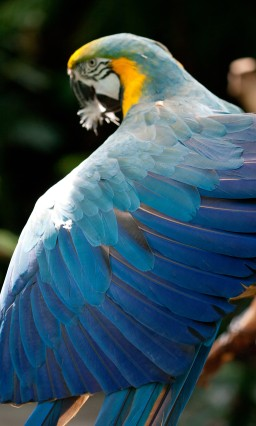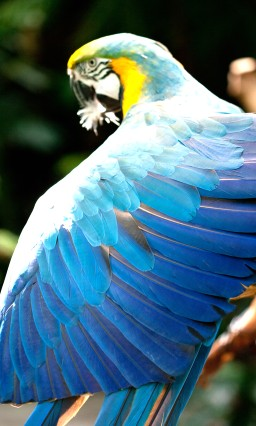```// Halide tutorial lesson 2: Processing images

// This lesson demonstrates how to pass in input images and manipulate
// them.

// On linux, you can compile and run it like so:
// g++ lesson_02*.cpp -g -I <path/to/Halide.h> -I <path/to/tools/halide_image_io.h> -L <path/to/libHalide.so> -lHalide `libpng-config --cflags --ldflags` -ljpeg -lpthread -ldl -o lesson_02 -std=c++17
// LD_LIBRARY_PATH=<path/to/libHalide.so> ./lesson_02

// On os x:
// g++ lesson_02*.cpp -g -I <path/to/Halide.h> -I <path/to/tools/halide_image_io.h> -L <path/to/libHalide.so> -lHalide `libpng-config --cflags --ldflags` -ljpeg -o lesson_02 -std=c++17
// DYLD_LIBRARY_PATH=<path/to/libHalide.dylib> ./lesson_02

// If you have the entire Halide source tree, you can also build it by
// running:
//    make tutorial_lesson_02_input_image
// in a shell with the current directory at the top of the halide
// source tree.

// The only Halide header file you need is Halide.h. It includes all of Halide.
#include "Halide.h"

#include "halide_image_io.h"
using namespace Halide::Tools;

int main(int argc, char **argv) {

// This program defines a single-stage imaging pipeline that
// brightens an image.

// First we'll load the input image we wish to brighten.

// See below for a smaller version.// Next we define our Func object that represents our one pipeline
// stage.
Halide::Func brighter;

// Our Func will have three arguments, representing the position
// in the image and the color channel. Halide treats color
// channels as an extra dimension of the image.
Halide::Var x, y, c;

// Normally we'd probably write the whole function definition on
// one line. Here we'll break it apart so we can explain what
// we're doing at every step.

// For each pixel of the input image.
Halide::Expr value = input(x, y, c);

// Cast it to a floating point value.
value = Halide::cast<float>(value);

// Multiply it by 1.5 to brighten it. Halide represents real
// numbers as floats, not doubles, so we stick an 'f' on the end
// of our constant.
value = value * 1.5f;

// Clamp it to be less than 255, so we don't get overflow when we
// cast it back to an 8-bit unsigned int.
value = Halide::min(value, 255.0f);

// Cast it back to an 8-bit unsigned integer.
value = Halide::cast<uint8_t>(value);

// Define the function.
brighter(x, y, c) = value;

// The equivalent one-liner to all of the above is:
//
// brighter(x, y, c) = Halide::cast<uint8_t>(min(input(x, y, c) * 1.5f, 255));
//
// In the shorter version:
// - I skipped the cast to float, because multiplying by 1.5f does
//   that automatically.
// - I also used an integer constant as the second argument in the
//   call to min, because it gets cast to float to be compatible
//   with the first argument.
// - I left the Halide:: off the call to min. It's unnecessary due
//   to Koenig lookup.

// Remember, all we've done so far is build a representation of a
// Halide program in memory. We haven't actually processed any
// pixels yet. We haven't even compiled that Halide program yet.

// So now we'll realize the Func. The size of the output image
// should match the size of the input image. If we just wanted to
// brighten a portion of the input image we could request a
// smaller size. If we request a larger size Halide will throw an
// error at runtime telling us we're trying to read out of bounds
// on the input image.
Halide::Buffer<uint8_t> output =
brighter.realize({input.width(), input.height(), input.channels()});

// Save the output for inspection. It should look like a bright parrot.
save_image(output, "brighter.png");

// See below for a small version of the output.printf("Success!\n");
return 0;
}
```